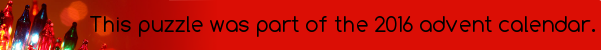mscroggs.co.uk
mscroggs.co.uksubscribe

# Puzzles

## 2 DecemberWhat is the maximum number of lines that can be formed by the intersection of 30 planes?

## Two lines

Let A and B be two straight lines such that the gradient of A is the y-intercept of B and the y-intercept of A is the gradient of B (the gradient and y-intercept of A are not the same). What are the co-ordinates of the point where the lines meet?

## Archive

Show me a random puzzle
▼ show ▼
© Matthew Scroggs 2012–2021